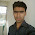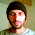Functions in TypeScript

In this post we are going to discuss about functions in TypeScript. As we all know that Functions are the fundamentals block of any applications in JavaScript. TypeScript functions are almost similar to JavaScript functions but there are different ways, how you can actually write functions in TypeScript.

There are mainly four ways how you can define a function in TypeScript and they are : Named Functions, Anonymous Functions, Lambda Functions and Class Functions.

1. Named functions

Actually this is similar to the function which we write in JavaScript. The only difference which you can find between Named TypeScript functions and Named JavaScript function is that, here we have a type on the parameter passed.The importance of having a type on the parameter is that you will only pass a parameter of that type (string in our case) when calling the function.

}

2. Anonymous Functions

The function is pretty much same to the Named Functions but here we will not be giving any name to the function. We will assign the function to a variable (square in our case). If we need to call the function we can simply write square(2). In our example below we are even assigning the return  type of the function that is number.

var square = function (a: number) : number {
return a * a;
}

3. Lambda functions

This function might be new to JavaScript developers, but it is not new to C# developers. Lets take an example to know about Lambda Functions.

var square = (a : number) => a * a; This is a actually a lambda function, where (a : number) is an inline parameter, (=>) is a lambda operator and (a * a) is the body of the function. When the above TypeScript code compiles it will give the below JavaScript code.

var square = function(a) { return a*a; }; Thus it is so easy to write a Lambda Function.

4. Class functions

This function is added inside a Class. The difference between the normal JavaScript functions and the functions written inside a Class is that you need not have to write a keyword 'function'.

class Square {
square(a: number) {
return a*a;
}
}

This above code will compile to the JavaScript shown below.

var Square = (function () {
function Square() {
}
Square.prototype.square = function (a) {
return a * a;
};
return Square;
})();

Please Like and Share the Blog, if you find it interesting and helpful.

1.2.3.